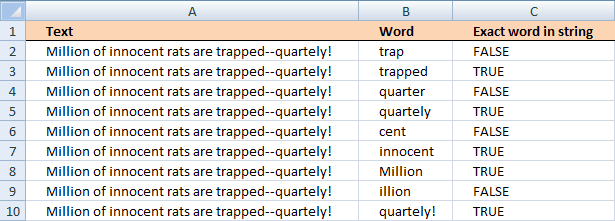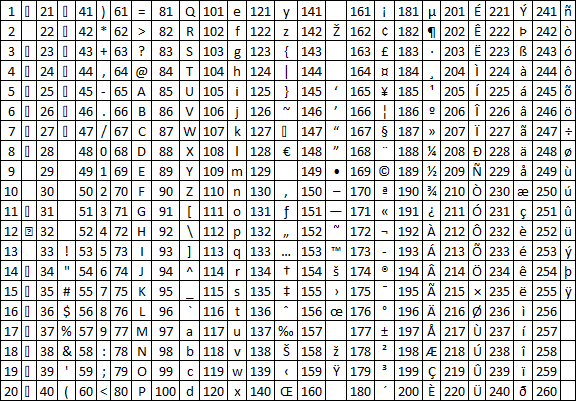Author: Oscar Cronquist Article last updated on November 23, 2018I read an interesting blog post Is A Particular Word Contained In A Text String? on Spreadsheetpage. That inspired me to create an excel formula doing the same thing as the vba function.

In short, from the above blog post

The function,  modified the first argument (Text) and replaces all non-alpha characters with a space character. It then adds a leading and trailing space to both arguments. Finally, it uses the Instr function to determine if the modified Word argument is present in the modified Text argument

The ExactWordInString function looks for a complete word -- not text that might be part of a different word.Edit: Read Rick Rothstein (MVP - Excel) comments at the bottom of this blog post.

Here is the formula I created in C2:

=IF(ISNUMBER(FIND(B2, A2)), IF(COUNT((IF(CODE(MID(A2, FIND(B2, A2)-1, 1))>122, 1, "")), (IF(CODE(MID(A2, FIND(B2, A2)-1, 1))<65, 1, "")), (IF(FIND(B2, A2)-1<1, 1, ""))), IF(COUNT((IF(CODE(MID(A2, FIND(B2, A2)+LEN(B2), 1))>122, 1, "")), (IF(CODE(MID(A2, FIND(B2, A2)+LEN(B2), 1))<65, 1, "")), (IF((FIND(B2, A2)+LEN(B2)+1)>LEN(A2), 1, ""))), TRUE, FALSE), FALSE), FALSE)

copied down as far as necessary.

### Explaining formula in cell C2

#### Step 1 - Check if string exists in cell

The FIND function returns the position of a string in cell value, if not found an error is returned. The ISNUMBER function returns TRUE for all numerical values and FALSE for all else even error values.

The IF function uses the boolean value to determine if the first argument or second argument is going to be returned.

IF(ISNUMBER(FIND(B2, A2)), formula, FALSE)

becomes

IF(ISNUMBER(FIND("trap", "Million of innocent rats are trapped--quartely!")), formula, FALSE)

becomes

IF(ISNUMBER(30), formula, FALSE)

becomes

IF(TRUE, formula, FALSE)

The IF function now continues the calculation with the first argument formula.

#### Step 2 - Check if the character before the string is larger than ansi code 122

The CODE function converts a character into ansi equivalent. Character "A" is 65 and "z" is 122.COUNT((IF(CODE(MID(A2,FIND(B2,A2)-1,1))>122,1,""))

becomes

COUNT((IF(CODE(MID(A2,FIND(("trap","Million of innocent rats are trapped--quartely!")-1,1))>122,1,""))

becomes

COUNT((IF(CODE(MID(A2,30-1,1))>122,1,""))

becomes

COUNT((IF(CODE(" ")>122,1,""))

becomes

COUNT((IF(32>122,1,""))

becomes

COUNT((IF(FALSE,1,""))

becomes

COUNT(("")

and returns 0.

#### Step 3 - Check if character before the string is less than ansi code 65

The MID function extracts a part of a string based on a start character and the length.

IF(CODE(MID(A2,FIND(B2,A2)-1,1))<65,1,"")

becomes

IF(CODE(MID(A2,30-1,1))<65,1,"")

becomes

IF(CODE(" ")<65,1,"")

becomes

IF(32<65,1,"")

and returns 1.

#### Step 4 - Check if string is at the very beginning

IF(FIND(B2,A2)-1<1,1,"")

becomes

IF(30-1<1,1,"")

becomes

IF(FALSE,1,"")

and returns "".

#### Step 5 - Check if the character after the string is larger than ansi code 122

The LEN function counts characters in a cell.

COUNT((IF(CODE(MID(A2,FIND(B2,A2)+LEN(B2),1))>122,1,""))

becomes

COUNT((IF(CODE("p")>122,1,""))

becomes

COUNT((IF(112>122,1,""))

becomes

COUNT((IF(112>122,1,""))

becomes

COUNT("")

and returns 0.

#### Step 6 - Check if character after the string is less than ansi code 65

The IF function returns a value determined by the logical expression in the first argument. If TRUE then the second argument is returned, FALSE returns the third argument.

IF(CODE(MID(A2,FIND(B2,A2)+LEN(B2),1))<65,1,"")

becomes

IF(CODE("p")<65,1,"")

becomes

IF(112<65,1,"")

and returns "".

#### Step 7 - Check if string is at the very end

IF((FIND(B2,A2)+LEN(B2)+1)>LEN(A2),1,"")

becomes

IF(35>LEN(A2),1,"")

becomes

IF(35>47,1,"")

and returns "".

#### Step 8 - Nested IFs

All these IF functions are nested.